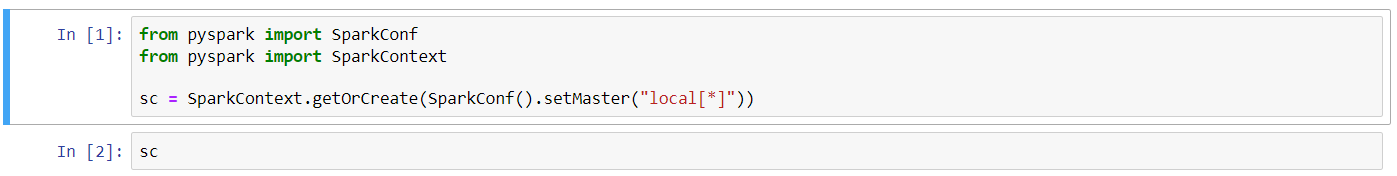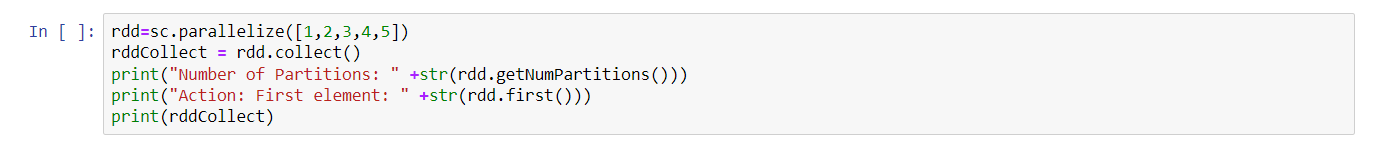# Spark

## Getting Started

Select Spark as the plugin.  There are no Application Parameters for this plugin. The plugin will open a Jypyter Notebook and a VNC instance on the selected system in seperate browser windows. Start a new notebook using the "new" tab.

Run these three short scripts to verify the Spark instance is connecting properly

1.

`from pyspark import SparkConf`
`from pyspark import SparkContext`
`sc = SparkContext.getOrCreate(SparkConf().setMaster("local[*]"))`

2.

`sc`image

3.

`rdd=sc.parallelize([1,2,3,4,5])`
`rddCollect = rdd.collect()`

`print("Number of Partitions: " +str(rdd.getNumPartitions()))`
`print("Action: First element: " +str(rdd.first()))`
`print(rddCollect)`image